﻿ 泛逻辑学理论——机制主义人工智能理论的逻辑基础
«上一篇文章快速检索 高级检索

 智能系统学报2018, Vol. 13Issue (1): 19-36  DOI: 10.11992/tis.2017110330

### 引用本文HE Huacan. Universal logic theory: logical foundation of mechanism-based artificial intelligence theory[J]. CAAI Transactions on Intelligent Systems, 2018, 13(1): 19-36. DOI: 10.11992/tis.201711033.### 文章历史

Universal logic theory: logical foundation of mechanism-based artificial intelligence theory
HE HuacanSchool of Computer Science, Northwestern Polytechnical University, Xi’an 710072, China
Abstract: Recently, the world’s major countries have begun to consider artificial intelligence (AI) as part of their national strategies. The widespread development of AI is changing our lives and ideas. In China, a small number of researchers have been concentrating their attentions onto the fundamental theory of intelligence based on dialectical materialism for more than 20 years, including the formation mechanism of intelligence, logical basis, mathematical foundation, coordination mechanism and contradiction transformation.At last, they set up the mechanism-based artificial intelligence theory, the universal logic theory, the factor space theory, the coordination theory, the extenics theory and the analysis of set pairs. The mechanism-based artificial intelligence theory is the general theory of intelligence based on the formation mechanism, it unifies the strucrualism, functionalism, and behaviorism, the existing three isolated major schools of artificial intelligence, into a harmonious unity, and makes the primary consciousness, emotion, and lntellect into a trinity; factor space theory is the mathematical foundation for mechanism-based artificial intelligence theory; universal logic theory is the logical based for mechanism-based artificial intelligence theory. In this paper, we introduces the basic theory and application of universal logic theory and clarifies its significance and value. Propositional universal logic (containing both hard and flexible logics) is based on " generalized probability theory”, and can be regarded as a proposition-level library of intelligent information processing operators. It contains all 18 flexible information processing modes (including 16 Boolean information processing modes). The operators are strictly expressed as $\left\langle {a,b,e} \right\rangle$ , so appropriate complete clusters of information processing operators can be identified. In each mode, different uncertainties are determined by the degree of encoding $\left\langle {k,h,\beta ,e} \right\rangle$ , which connects with the direct operator required. Consequently, flexible information processing can considered to be a coded lock, with $\left\langle {{a,b,e}} \right\rangle + \left\langle {k,h,\beta ,e} \right\rangle$ as its passwords. Additionally, all of the passwords are saved in the library, thereby satisfying the demand for " one lock-one passwords”.
Key words: universal logic    mechanism-based artificial intelligence theory    uncertainty    flexible logic    intelligent information processing operator library

1 研究背景 1.1 人工智能的理论危机

20世纪中叶人工智能学科的诞生开创了智能信息处理的新纪元。众所周知，各种人工智能学派都是在布尔信息处理和标准逻辑(数理逻辑)基础上建立和发展起来的，20世纪80年代的人工智能理论危机暴露了布尔信息处理和标准逻辑的应用局限性[2-3]

1)由于布尔信息处理和标准逻辑只是根据命题真值而不考虑命题内容进行的推理，尽管它具有可机械执行，无需领域背景知识支撑的优点，但在机械执行过程中也带来了工作效率十分低下、无法克服因信息处理算法的时空复杂度带来的组合爆炸的缺点；

2)由于布尔信息处理和标准逻辑只考虑了确定性推理的需要，必须满足非此即彼的理想化约束。而智能信息处理需要面对的现实问题往往具有各种不确定性，推理的各种逻辑要素常常有信息缺损和不断变化的情况，不允许像传统数学问题那样进行理想化处理，带有亦此亦彼性特征。对于这类问题，布尔信息处理和标准逻辑束手无策，无所适从。

1.2 两种不同的发展倾向

1)尽可能回避甚至放弃人工智能的逻辑主义路线，大力发展非逻辑的结构主义(人工神经网络)和行为主义(刺激—反应)路线。在这一发展倾向下，近几十年来基于大数据处理的深度神经网络、各种计算智能、群体智能和机器学习方法有了长足的进步，取得了举世瞩目的应用成果。

2)继续坚持逻辑主义路线，并针对处理各种不确定性的需要，近几十年来涌现出了几十种非标准逻辑和一批不确定性推理理论，它们虽然可满足某些智能信息处理的应用需求，但有时会出现无法容忍的反常结果，这表明这些非标准逻辑和不确定性推理方法在理论上并不成熟，人们还没有精确掌握其立论依据和有效使用范围。

1.3 作者的个人选择Download: 图 1 从概率论看3个非标准逻辑适用的状态点 Fig. 1 See the state point of application of 3 nonstandard logic from the theory of probability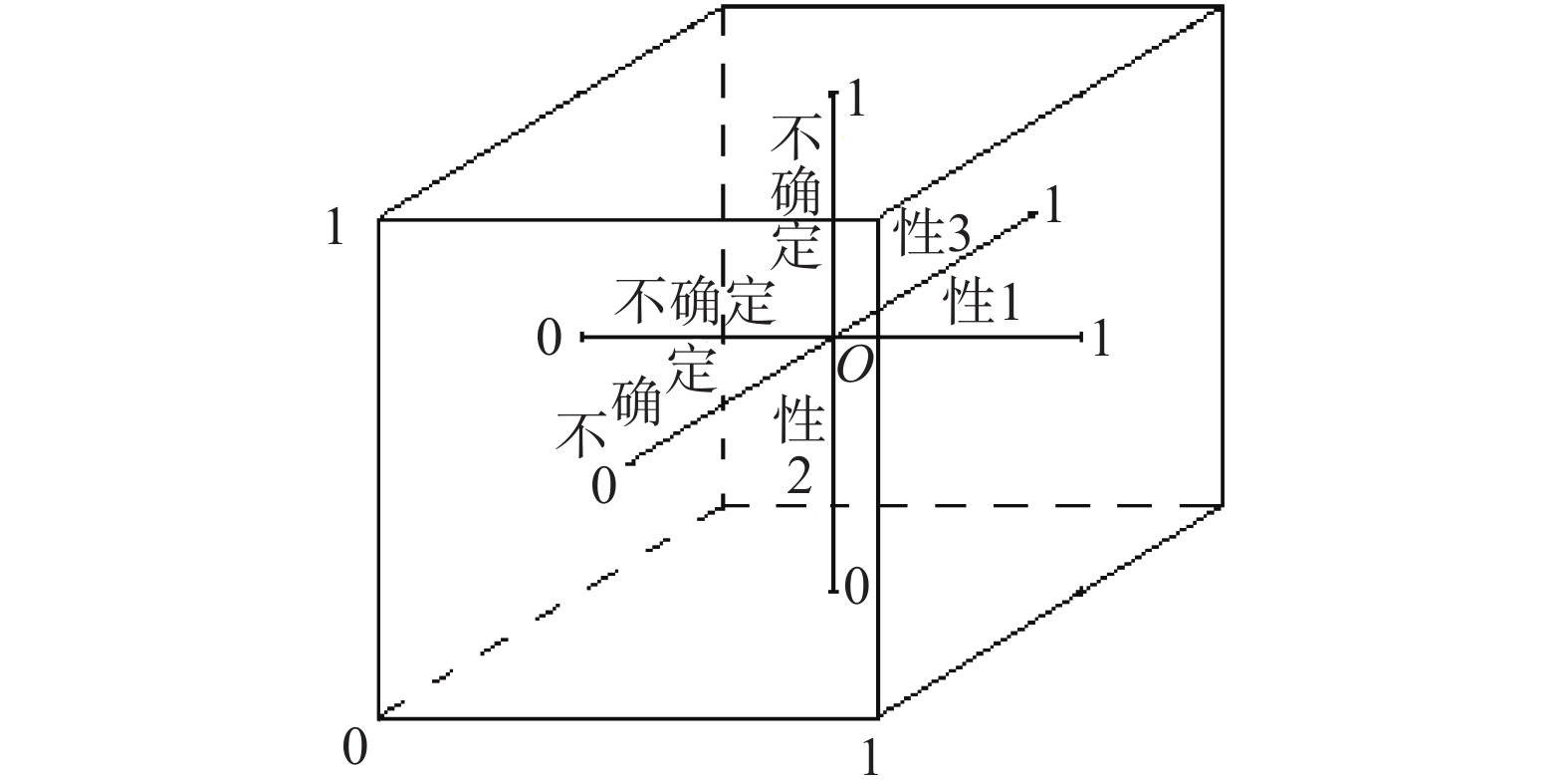Download: 图 2 命题泛逻辑的理论框架 Fig. 2 Theoretical framework for propositional universal logic

1.4 寻找数学基础理论支撑和广泛的应用检验

1)概率论中的相关系数只考虑了两个命题(事件)之间的相容(相生)性相关关系，即从最大相吸相关关系开始，不断向下连续变化到独立相关关系，再不断向下连续变化到最大相斥相关关系。我们引入的广义相关系数已全面考虑了两个命题(事件)之间的相容(相生)性相关和不相容(相克)性相关，即增加了从最大相斥相关关系继续向下连续变化到不同程度的冷战关系，再向下连续变化到不同程度的热战关系，这种相关性扩张更加符合描述客观存在的各种相关关系的需要。

2)在概率论中，原来只给出了最大相吸相关、独立相关和最大相斥相关3个特殊点的逻辑运算公式组，对三点之间连续分布的其他点的逻辑运算公式组没有给出，是用条件概率计算公式来代替的。我们在广义概率论中，把广义相关系数中的每一个点的逻辑运算公式组全都给出了，没有一点遗漏，大大方便了不确定性推理。

2 关于智能信息处理的哲学思考 2.1 确定论宇宙观是不确定论宇宙观在局部时空中的特例

2.2 传统数学问题与智能信息处理的差别

2.3 应该关注智能系统的时效性

2.4 传统数学问题为何能用刚性推理范式求解

2.5 智能信息处理为何必须用柔性推理范式求解

2.6 在智能信息处理中需要两种推理范式同时并存各司其职

2.7 有关智能信息处理的若干基本假设

2.8 小结Download: 图 3 智能信息处理对逻辑学的需求 Fig. 3 The need for intelligent information processing to logic
3 命题泛逻辑的研究目标和研究基础 3.1 命题泛逻辑的研究目标

3.2 布尔信息处理的有关情况

1)基于逻辑推理法的布尔信息处理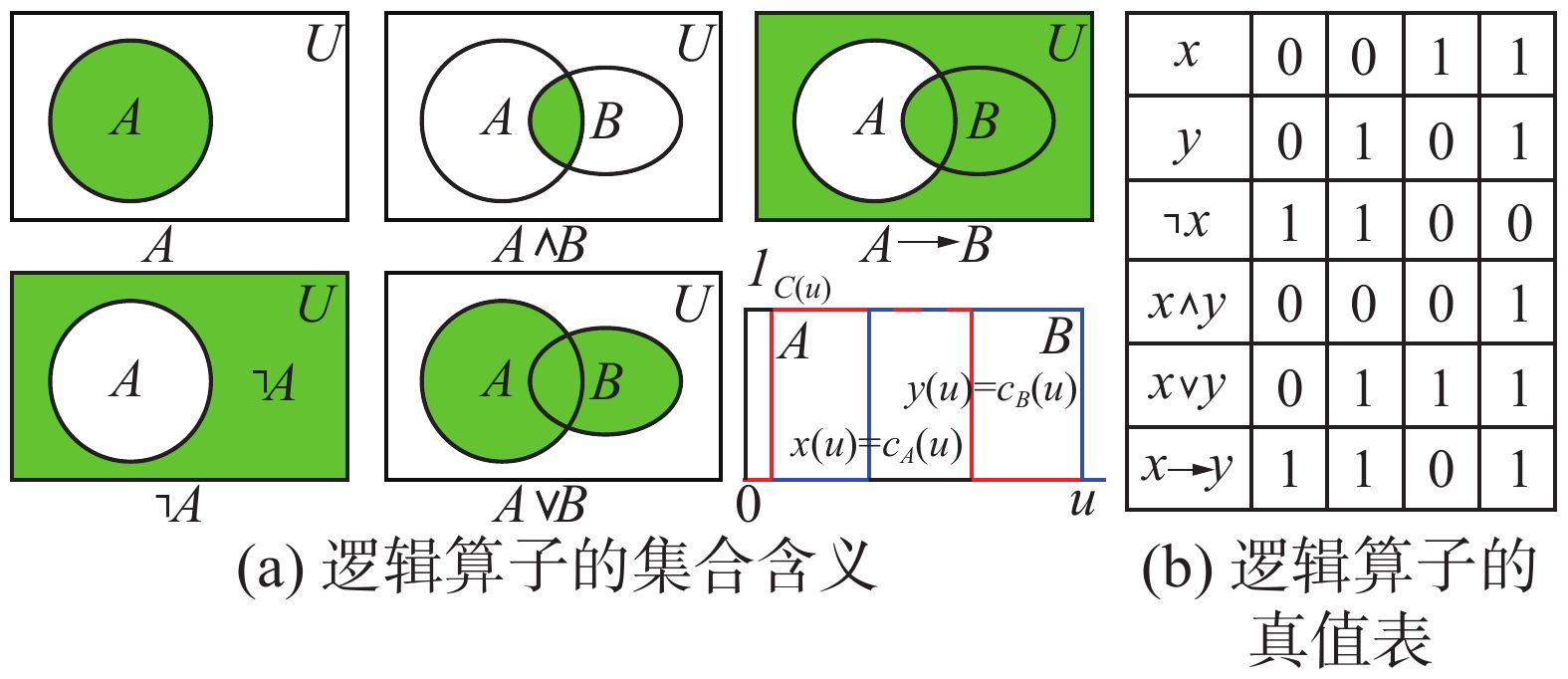Download: 图 4 布尔逻辑算子组及其在概率论中的集合意义 Fig. 4 Boolean logic operator group and its set meaning in probability theory

2)基于神经网络信息变换法的布尔信息处理

1943年心理学家McCulloch和数学家W. Pitts提出二值神经元信息变换模型 ${\rm{M}} - {\rm{P}}$ (又称感知机)图5是二值神经元的基本结构，其中 $x,y,z \in \left\{ {\left. {0,1} \right\}} \right.$ , $a{\text{、}}b$ 是输入权系数， $e$ 是反应阈值， $v = ax +$ $by - e$ 是整合函数，经 $\Gamma \left[ v \right]$ 进行0、1限幅处理后输出 $z$ ，结果只有0或1状态，每一次信息处理都有 $\Delta {\rm{t}}$ 的延迟。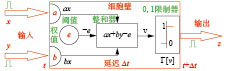Download: 图 5 二值神经元M-P模型的基本结构 Fig. 5 The basic structure of the two value neuron M-P model

3)布尔信息处理的完备性分析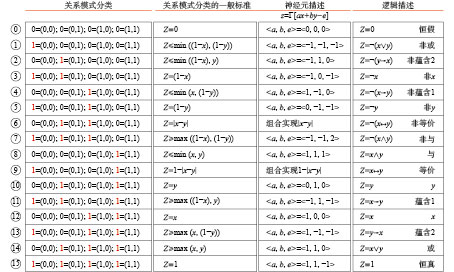Download: 图 6 布尔信息处理的完备性分析 Fig. 6 Completeness analysis of Boolean information processing

4)布尔信息处理的最基本模式分析

3.3 连续值信息处理的有关情况

 $\begin{array}{c}x \wedge y = \min \left( {x,y} \right) = xy = \Gamma \left[ {x + y - 1} \right] = \\{\mathop{\rm ite}\nolimits} \left\{ {\left. {\left. {\min \left( {x,y} \right)} \right|\max \left( {x,y} \right) = 1;0} \right\}} \right.\\x \vee y = \max \left( {x,y} \right) = x + y - xy = \Gamma \left[ {x + y} \right] = \\{\mathop{\rm ite}\nolimits} \left\{ {\left. {\left. {\max \left( {x,y} \right)} \right|\min \left( {x,y} \right) = 0;1} \right\}} \right.\\x \to y = {\mathop{\rm ite}\nolimits} \left\{ {\left. {\left. 1 \right|x \leqslant y;y} \right\}} \right. = \min \left( {1,x/y} \right) = \\\Gamma \left[ {1 - x + y} \right] = {\mathop{\rm ite}\nolimits} \left( {\left. y \right|x = 1;1} \right)\end{array}$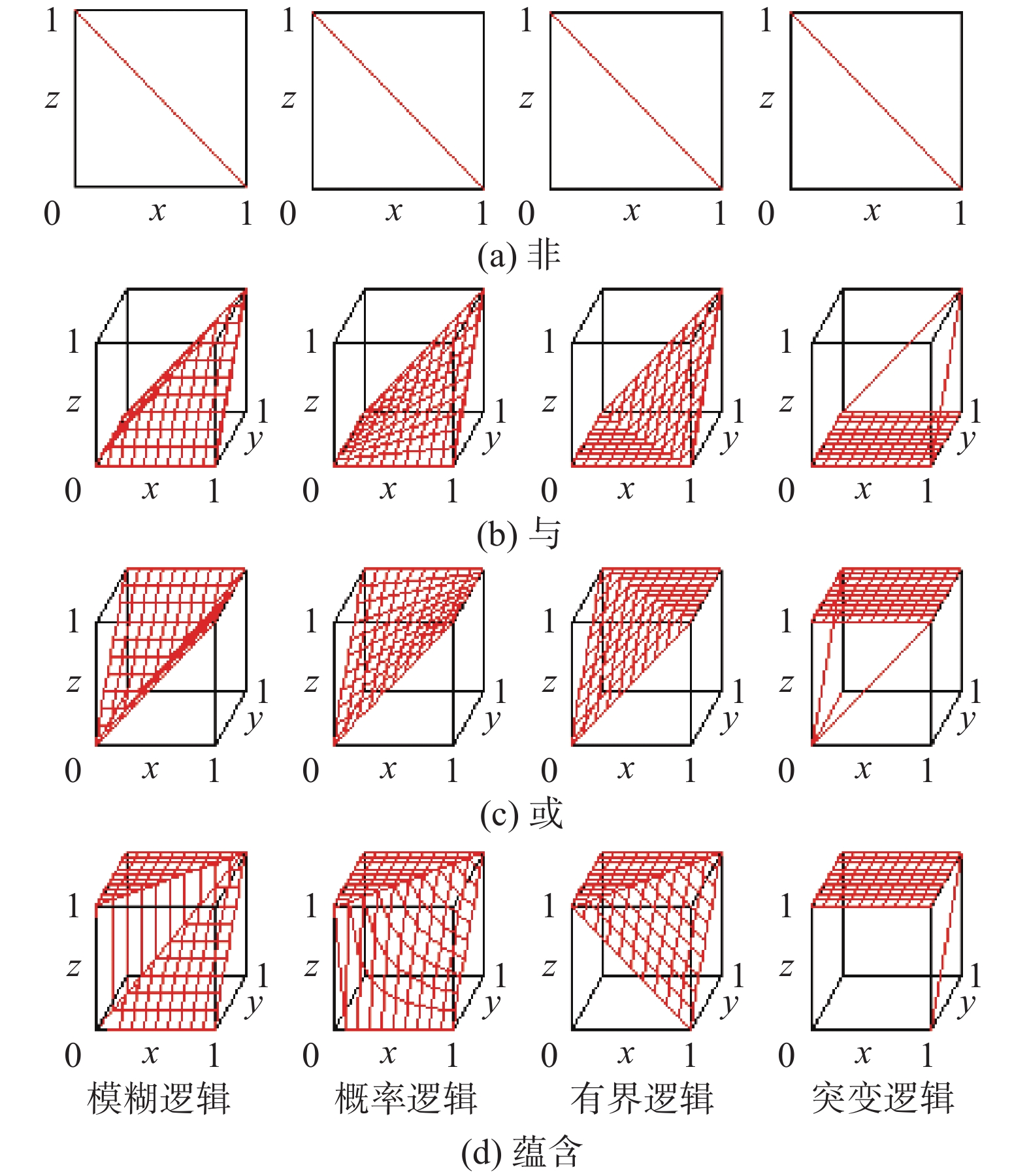Download: 图 7 4个非标准逻辑引发的思考 Fig. 7 Thinking caused by 4 nonstandard logic

 $\begin{array}{c}\neg x = 1 - x;x \wedge y = \min \left( {x,y} \right);x \vee y = \max \left( {x,y} \right);\\x \to y = {\mathop{\rm ite}\nolimits} \left\{ {\left. {\left. 1 \right|x \leqslant y;y} \right\}} \right.\end{array}$

 $\begin{array}{c}\neg x = 1 - x;x \wedge y = xy;x \vee y = x + y - xy;\\x \to y = \min \left( {1,x/y} \right)\end{array}$

 $\begin{array}{c}\neg x = 1 - x;x \wedge y = \Gamma \left[ {x + y - 1} \right];\\x \vee y = \Gamma \left[ {x + y} \right];x \to y = \Gamma \left[ {1 - x + y} \right]\end{array}$

 $\begin{array}{c}\neg x = 1 - x;x \wedge y = {\mathop{\rm ite}\nolimits} \left\{ {\left. {\left. {\min \left( {x,y} \right)} \right|\max \left( {x,y} \right) = 1;0} \right\}} \right.;\\x \vee y = {\mathop{\rm ite}\nolimits} \left\{ {\left. {\left. {\max \left( {x,y} \right)} \right|\min \left( {x,y} \right) = 0;1} \right\}} \right.;\\x \to y = {\mathop{\rm ite}\nolimits} \left( {\left. y \right|x = 1;1} \right)\end{array}$

3.4 三角范数理论的启发

1942年K. Menge提出三角范数(triangular norm)概念，主要研究各种算子中不同运算模型应共同满足的抽象定义、一般性质和生成方法，常用的连续值域为 $\left[ {0,1} \right]$ 。根据三角范数理论的研究，上述4个连续值逻辑全部包含于Schweizer算子簇 ${x^m},m \in \left( { - \infty ,\infty } \right)$ 中。概率论只孤立发现了前3个逻辑点 $\left( {m = - \infty ,0,1} \right)$ ，而Schweizer 算子簇则包含了连续区间 $m \in \left( { - \infty ,\infty } \right)$ 中的所有逻辑点，其中包括突变逻辑点 $m = \infty$ ，以及在这4个特殊逻辑点空隙中间存在的所有逻辑点(见图8)。由此可以看出，智能信息处理可以利用Schweizer算子簇将概率论进一步扩张完善，而且这个扩张完善的空间很大！这一发现给了我们深入探索下去的勇气，并有了得心应手的数学工具。

4 智能信息处理的理论依据是广义概率论 4.1 柔性命题的真度是它在因素空间中对应集合的概率测度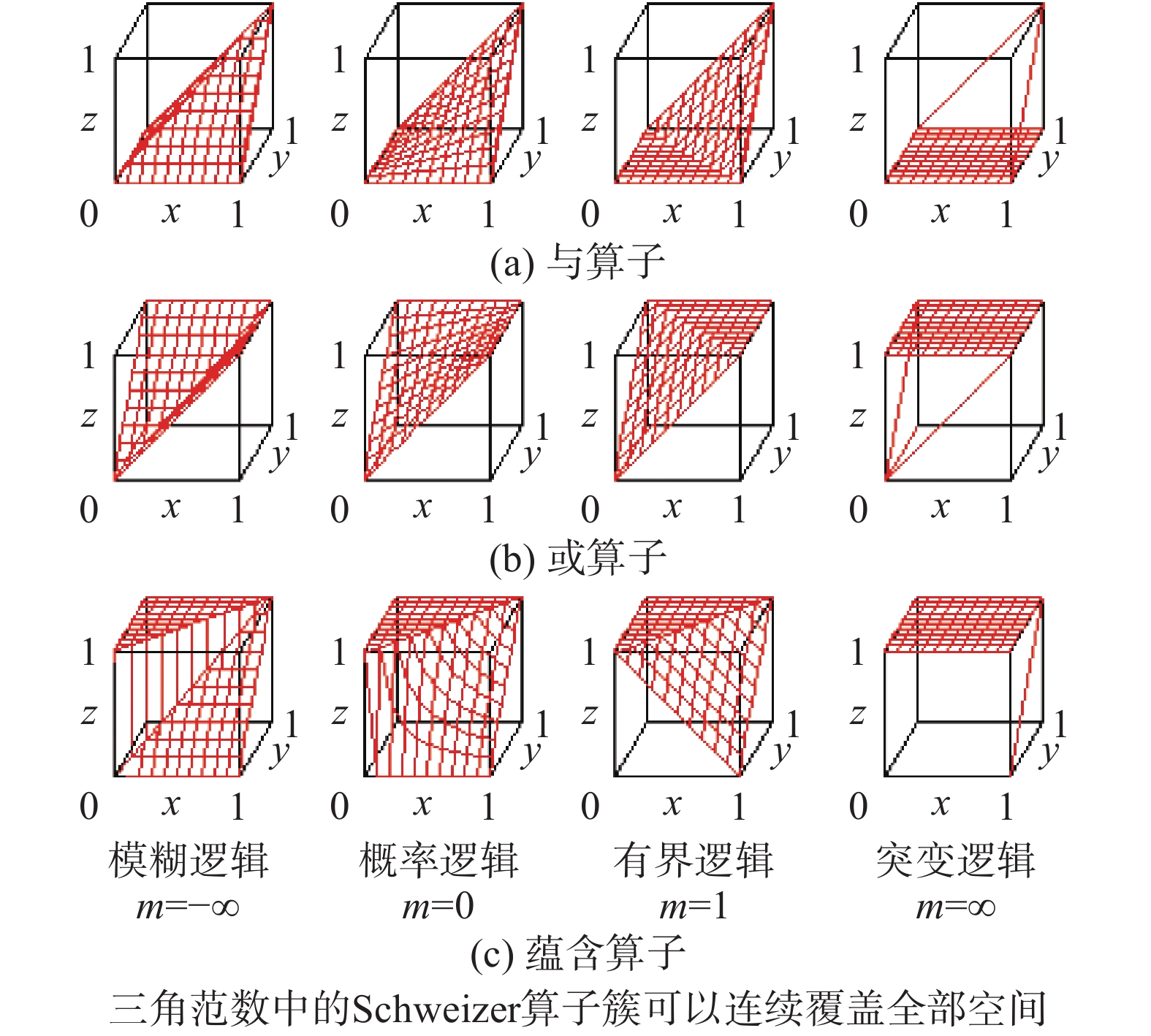Download: 图 8 用Schweizer算子簇扩张概率论 Fig. 8 Extension probability theory with Schweizer operater cluster

1)柔性命题真度的确定方法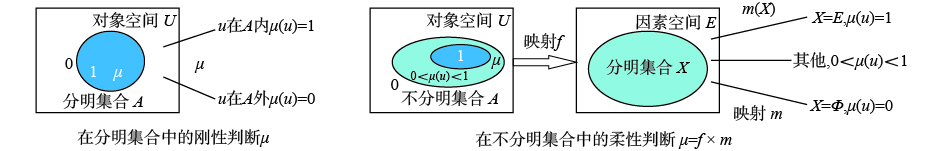Download: 图 9 柔性命题真度的定义 Fig. 9 The definition of the truth degree of the flexible propositions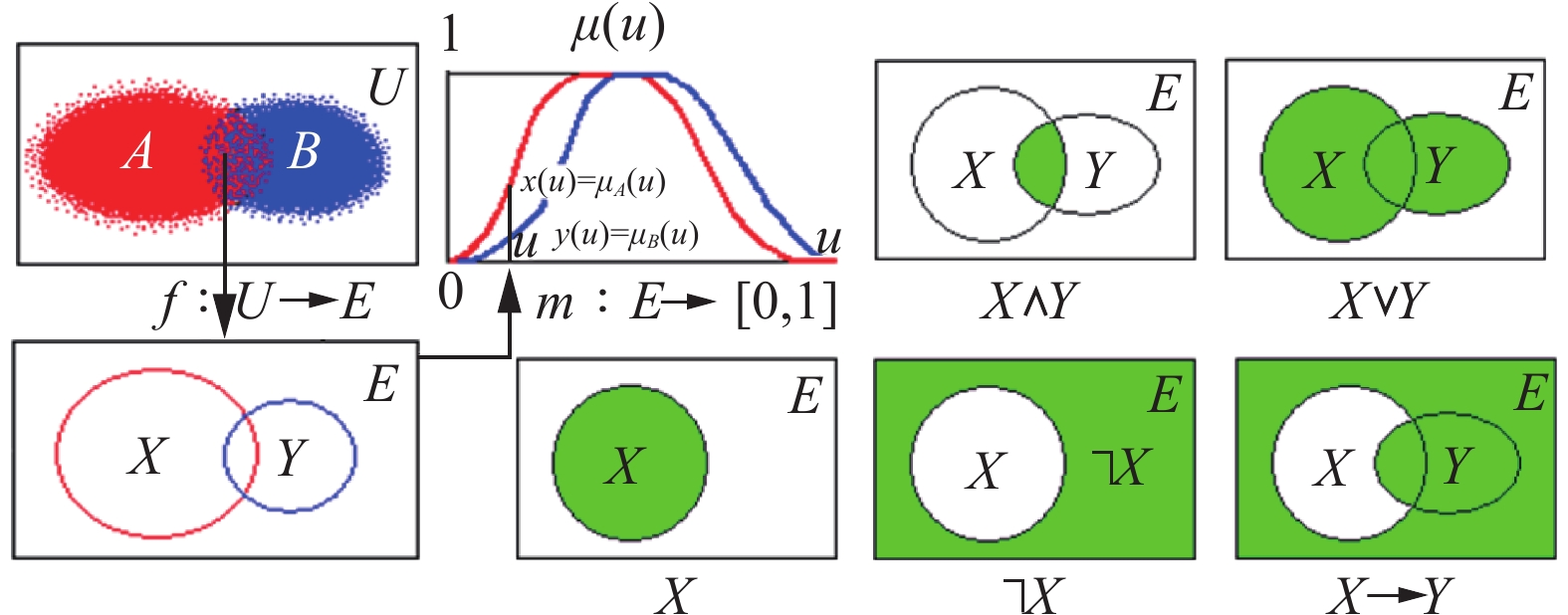Download: 图 10 柔性命题真度的确定方法 Fig. 10 A method for determining the truth degree of flexible propositions

2) 两级间接定义的方法在智能信息处理中具有普适性意义

4.2 命题真度变化对各种逻辑运算的影响表现在它的基模型上

1) 18种不同的柔性信息处理模式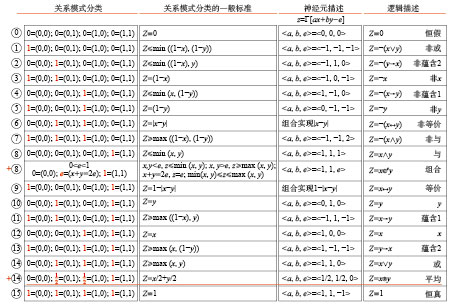Download: 图 11 18种柔性信息处理模式 Fig. 11 Eighteen kinds of flexible information processing mode

2) 常用的7种基模型

①非运算的基模型　 $\neg x = N\left( x \right) = 1 - x$

②与运算的基模型　 $x \wedge y = T\left( {x,y} \right) = \Gamma \left[ {x + y - 1} \right]$

③或运算的基模型　 $x \vee y = S\left( {x,y} \right) = \Gamma \left[ {x + y - 1} \right]$

④蕴含运算的基模型　 $x \to y \!=\! I\left( {x,y} \right) \!=\! \Gamma \left[ {1 \!-\! x \!+\! y} \right]$

⑤等价运算的基模型　 $x \leftrightarrow y \!=\! Q\left( {x,y} \right) \!=\! 1 \!-\! \left| {x \!-\! y} \right|$

⑥平均运算的基模型　 x®y = $M(x,y) \!=\! {\rm{1 \! -\! [\{ (1 \! - \! x)/ }}$ $2 + {\rm{(1 - y)/2\} ]}}$

⑦组合运算的基模型　 x©y $\!= \!{C^e}(x,y) \!=\! \Gamma [x \!+\! y \!- \!0.5]$

4.3 破译不确定性推理的密码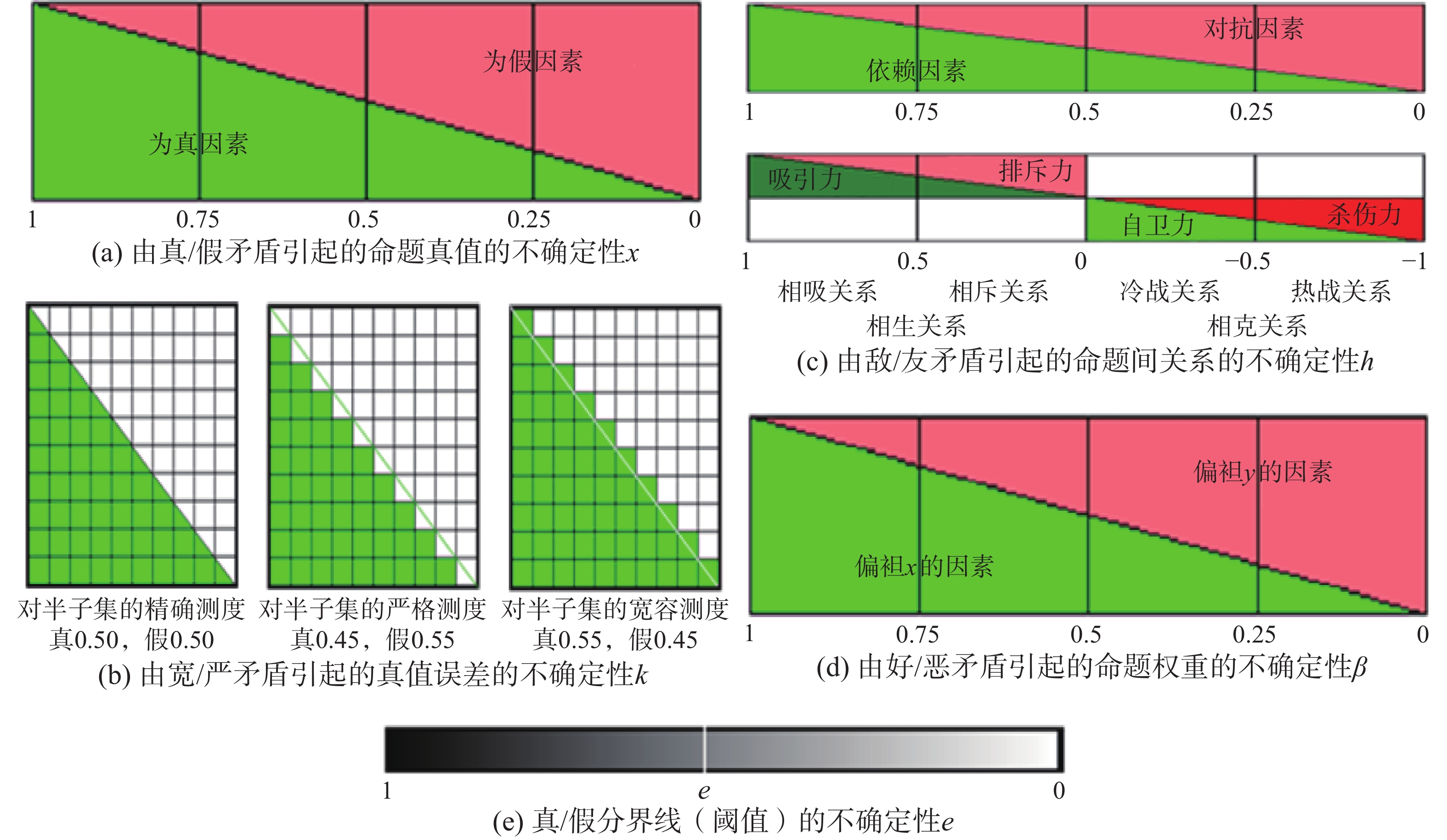Download: 图 12 命题泛逻辑中能够包容的5种不确定性 Fig. 12 Five kinds of uncertainty that can be contained in propositional universal logic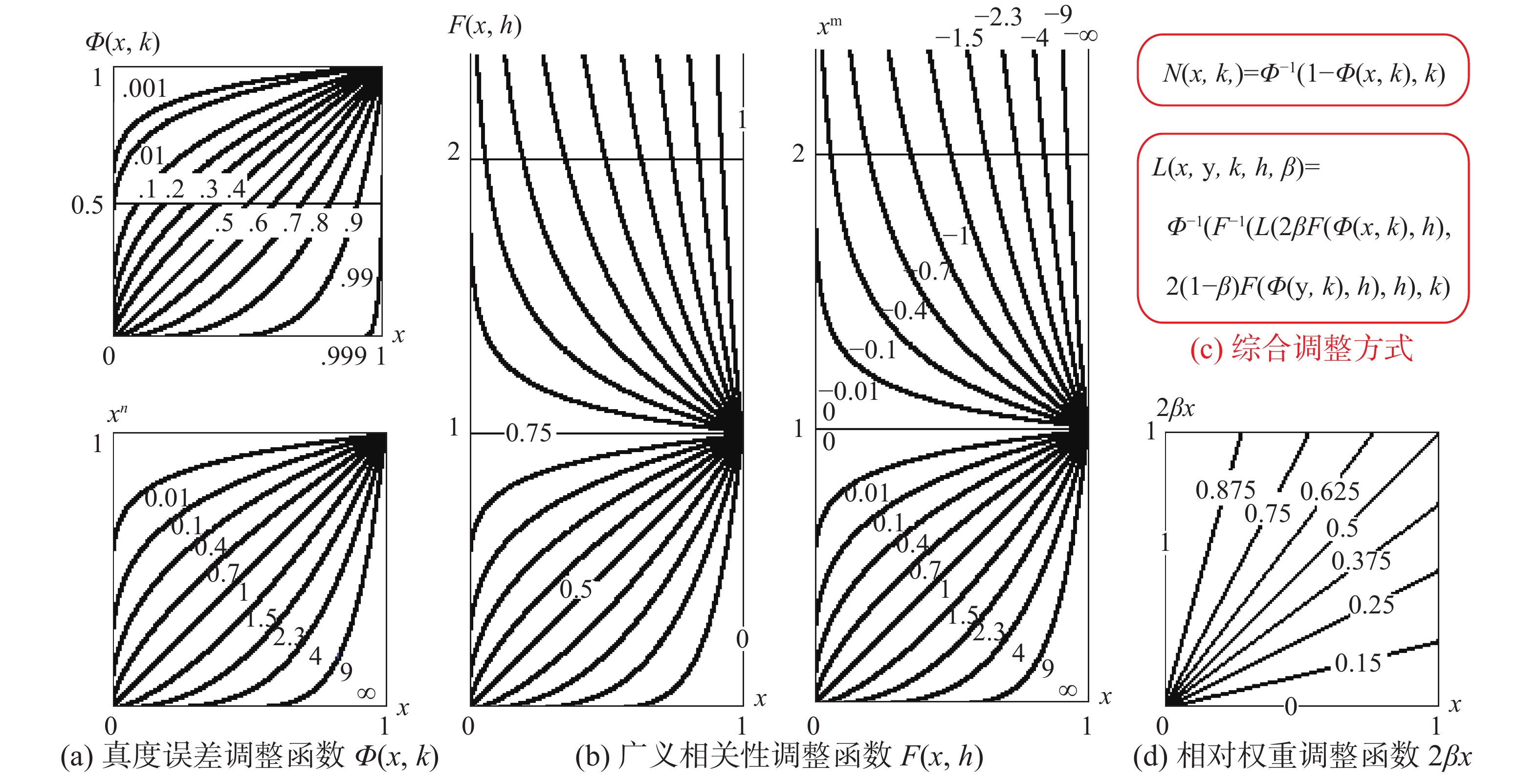Download: 图 13 各种不确定性对基模型组的调整函数 Fig. 13 The adjustment function of a variety of uncertainty to the base model group

1)命题真度误差的不确定性

 $N\left( {x,k} \right) = {\varPhi ^{ - 1}}\left( {N\left( {\varPhi \left( {x,k} \right)} \right),k} \right)$

 $L\left( {x,y,k} \right) = {\varPhi ^{ - 1}}\left( {L\left( {\varPhi \left( {x,k} \right),\varPhi \left( {y,k} \right)} \right),k} \right)$

2) 两个命题之间广义相关关系的不确定性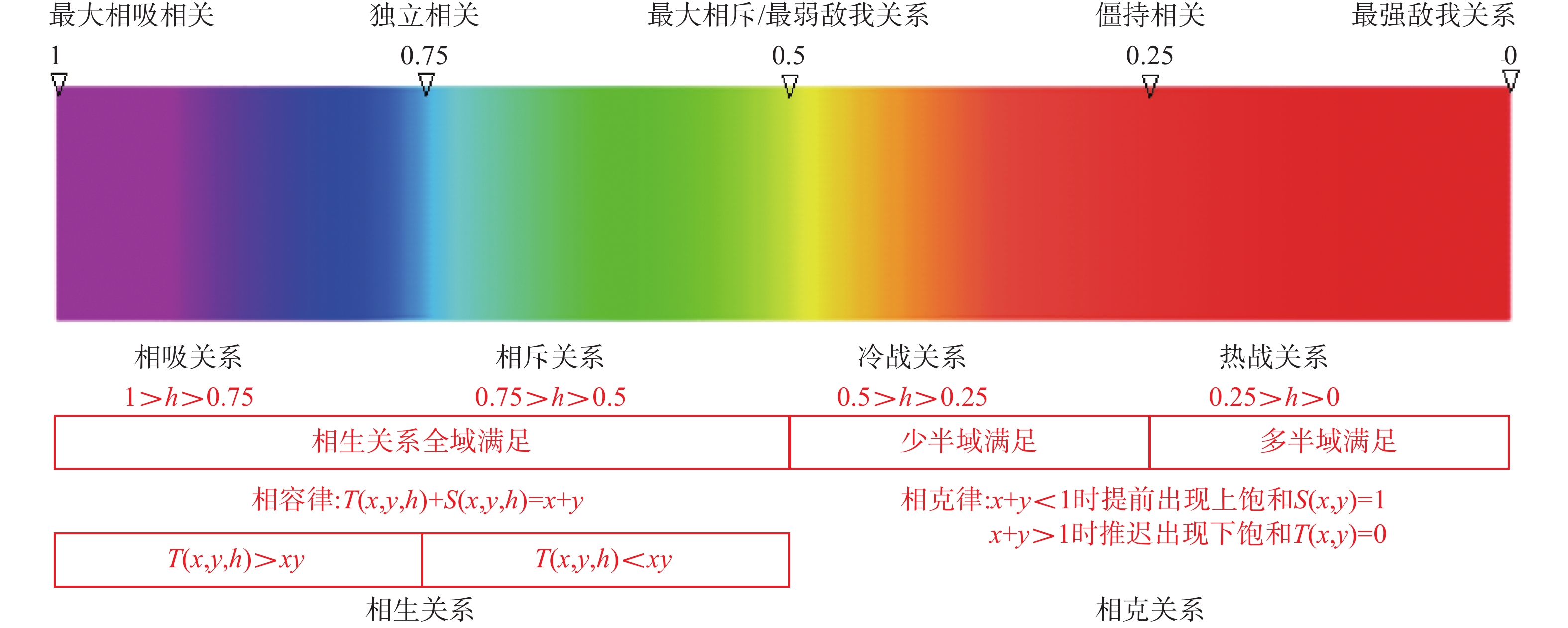Download: 图 14 柔性广义相关关系的不确定性 Fig. 14 Uncertainty of flexible generalized correlation

$h \in \left[ {0,0.5} \right)$ 是敌我关系的逻辑抽象，必然发生相互损伤和过分消耗资源的行为，其数学特征满足相克律：当x+y<1时，会提前出现上饱和效应，S(x, y, h)=1; 当x+y>1时，会推迟出现下饱和效应，T(x, y, h)=0。其中T(x, y, 0.25)=0和S(x, y, 0.25)=1的机会正好是一半一半，所以h=0.25点代表的是僵持关系；当h>0.25时，T(x, y, h)=0和S(x, y, h)=1的机会小于一半甚至趋近于0，代表的是冷战关系，主要是因为扩军备战和储备战略资源；当h<0.25时，T(x, y, 0)=0和S(x, y, 0)=1的机会大于一半甚至趋近于1，代表的是热战关系，主要是因为人员的大量伤亡和物资的大量消耗。到了h=0时，突然变成T(x, y, 0)=ite{min(x, y)|max(x, y)=1; 0}和S(x, y, 0)= ite{max(x, y)|min(x, y)=0; 1}，代表敌人已经完全消灭，胜利一方已经控制了一切。这些都是特别重要的逻辑特征点。

$L(x,y,h) = {F^{ - 1}}(L(F(x,h),F(y,h)),h)$

3) 两命题之间相对权重的不确定性

 $L(x,y,\beta ) = L(2\beta x,{\rm{ }}2(1 - \beta )y)$

4) 组合运算中决策阈值的不确定性

 $\begin{array}{c}{C^e}\left( {x,y} \right) = {\rm{ite}}\{ {\rm{min}}\left( {e,\max \left( {0,x + y - e} \right)} \right)|x + y < 2e;\\N\left( {{\rm{min}}\left( {N\left( e \right),{\rm{max}}\left( {0,N\left( x \right) + N\left( y \right) - N\left( e \right)} \right)} \right)} \right)|\\x + y{\rm{ > }}2e;e\} = {\rm{min}}\left( {1,{\rm{max}}\left( {0,x + y - e} \right)} \right)\end{array}$

4.4 7种逻辑运算的公理及模型

1)非运算公理及模型

 $N(x,k){\rm{ }} = {\varPhi ^{ - 1}}(1 - \varPhi (x,k),k){\rm{ }} = {\rm{ }}{(1 - {x^n})^{1/}}^n$

2)与运算公理及模型

$T(x,y,k,h,\beta ) = {({\rm{max}}(0,{\rm{ }}2\beta {x^{nm}} + 2(1 - \beta ){y^{nm}} - 1))^{1/}}^{mn}$

$\beta = 0.5$ 时，偏袒性的影响消失， $T(x,y,k,h) = ({\rm{max}}(0,$ ${{x^{nm}} +y^{nm}} - 1){)^{1/}}^{mn}$ ；当 $k = 0.5$ 时，误差的影响消失， $T(x,y,h) = {\rm{ }}{({\rm{max}}(0,{x^m} + {y^m} - 1))^{1/}}^m$

$T\left( {x,y,{\rm{ }}0} \right) = \operatorname{ite} \left\{ {{\rm{min}}\left( {x,y} \right)|{\rm{max}}\left( {x,y} \right) = 1;{\rm{ }}0} \right\}$

3) 或运算公理及模型

 $\begin{array}{c}S\left( {x,y,k,h,\beta } \right) = \\{\left( {1 - {{\left( {{\rm{max}}\left( {0,2\beta {{\left( {1 - {x^n}} \right)}^m} + 2\left( {1 - \beta } \right){{\left( {1 - {y^n}} \right)}^m} - 1} \right)} \right)}^{1/m}}} \right)^{1/n}}\end{array}$

$\, \beta = 0.5$ 时，偏袒性的影响消失， $S\left( {x,y,k,h} \right) =$ ${\left( {1 - {{\left( {{\rm{max}}\left( {0,{{\left( {1 - {x^n}} \right)}^m} + {{\left( {1 - {y^n}} \right)}^m} - 1} \right)} \right)}^{1/m}}} \right)^{1/n}}$ ；当 $k = 0.5$ 时，误差的影响消失， $S\left( {x,y,h} \right) = \left( {1 - \left( {{\rm{max}}\left( {0,{{\left( {1 - x} \right)}^m} + } \right.} \right.} \right.$ ${\left( {1 - y} \right)^m} - 1)){)^{^{1/m}}}$ $S\left( {x,y,h} \right)$ 有4个特殊算子:模糊或算子 $S\left( {x,y,{\rm{ }}1} \right) = {\rm{max}}\left( {x,y} \right)$ ，概率或算子 $S\left( {x,y,{\rm{ }}0.75} \right) = x +$ y – xy，有界或算子 $S\left( {x,y,{\rm{ }}0.5} \right) = {\rm{min}}\left( {1,x + y} \right)$ ，突变或算子 $S\left( {x,y,{\rm{ }}0} \right) = {\rm{ite}}\left\{ {{\rm{max}}\left( {x,y} \right)|{\rm{min}}\left( {x,y} \right) = 0;1} \right\}$

$S\left( {x,y,k,h} \right)$ $T\left( {x,y,k,h} \right)$ 之间存在对偶律： $N\left( {S\left( {x,y,k,h} \right),k} \right) \!=\! T\left( {N\left( {x,k} \right),N\left( {y,k} \right),k,h} \right)$ $N( {T\left( {x,y,k,h} \right),}$ $S k) =\left( {N\left( {x,k} \right),N\left( {y,k} \right),k,h} \right)$ ，当 $h \in \left[ {0.5,{\rm{ }}1} \right]$ 时, $S\left( {x,y,h} \right)$ $T\left( {x,y,h} \right)$ 满足相容律 $S\left( {x,y,h} \right) + T\left( {x,y,h} \right) = x + y$

4) 蕴涵运算公理及模型

$I\left( {x,y,k,h,\beta } \right) = {\left( {{\rm min}\left( {1,1 - 2\beta {x^{nm}} + 2\left( {1 - \beta } \right){y^{nm}}} \right)} \right)^{1/mn}}$

$\beta = 0.5$ 时，偏袒性的影响消失， $I\left( {x,y,k,h} \right) =$ ${({\rm{min}}(1,1 - x^{nm}} + {y^{nm}}){)^{^{1/mn}}}$ ；当 $k = 0.5$ 时，误差的影响消失， $I\left( {x,y,h} \right) = {\left( {{\rm{min}}\left( {1,1 - {x^m} + {y^m}} \right)} \right)^{1/m}}$

$I\left( {x,y,h} \right)$ 有4个特殊算子: 模糊蕴涵 $I\left( {x,y,1} \right) =$ ${\rm{ite}}\left\{ {1|x \leqslant y;y} \right\}$ ，概率蕴涵 $I\left( {x,y,0.75} \right) = \min \left( {1,y/x} \right)$ ，有界蕴涵 $I\left( {x,y,0.5} \right) = {\rm{min}}\left( {1,1 - x + y} \right)$ ，突变蕴涵 $I\left( {x,y,0} \right) =$ $\operatorname{ite} \left\{ {y|x = 1;1} \right\}$

5)等价运算公理及模型

 $\begin{array}{c}{Q(x,y,k,h,\beta ) = }\\{{\rm{ite}}\left\{ {\left. {{{\left( {1 + \left| {2\beta {x^{nm}} - 2\left( {1 - \beta } \right){y^{nm}}} \right|} \right)}^{1/mn}}} \right|} \right\}}\\\left. {m \leqslant 0;{{\left( {1 - \left| {2\beta {x^{nm}} - 2\left( {1 - \beta } \right){y^{nm}}} \right|} \right)}^{1/mn}}} \right\}\end{array}$

$\beta = 0.5$ 时，偏袒性的影响消失， $Q\left( {x,y,k,h} \right) =$ ${\rm{ite}}\left\{ {{{\left( {1 + \left| {{x^{nm}} - {y^{nm}}} \right|} \right)}^{\frac{1}{{mn}}}}\left| {m \leqslant 0} \right.;{\rm{ }}{{\left( {1 - \left| {{x^{nm}} - {y^{nm}}} \right|} \right)}^{\frac{1}{{mn}}}}} \right\}$ ；当 $k =$ 0.5时，误差的影响消失， $Q\left( {x,y,h} \right) \!\!=\!\! {\rm{ite}}\left\{ {\left. {{{\left( {1 \!\!+\!\! \left| {{x^m} \!-\! {y^m}} \right|} \right)}^{1/m}}} \right|m0;} \right.$ $\left. {{{\left( {1 - \left| {{x^m} - {y^m}} \right|} \right)}^{1/m}}} \right\}$

$Q\left( {x,y,h} \right)$ 有4个特殊算子:模糊等价 $Q\left( {x,y,1} \right) =$ ${\rm{ite}}\left\{ {1|x = y;{\rm{min}}\left( {x,y} \right)} \right\}$ ，概率等价 $Q\left( {x,y,0.75} \right) =$ ( ${\rm{I}}$ 等价)，有界等价 $Q\left( {x,y,0.5} \right) = 1 - \left| {x - y} \right|$ ( ${\rm{S}}$ 等价)，突变等价 $Q\left( {x,y,0} \right) = {\rm{ite}}\left\{ {x|y = 1;\;y|x = 1;1} \right\}$

6)平均运算公理及模型

$M\left( {x,y,k,h,\beta } \right) = {\left( {1 - {{\left( {\beta {{\left( {1 - {x^n}} \right)}^m} + \left( {1 - \beta } \right){{\left( {1 - {y^n}} \right)}^m}} \right)}^{1/m}}} \right)^{1/n}}$

$\beta = 0.5$ 时，偏袒性的影响消失， $M\left( {x,y,k,h} \right) =$ ${\left( {1 - {{\left( 0.5{{{\left( {1 - {x^n}} \right)}^m} + 0.5 {{\left( {1 - {y^n}} \right)}^m}} \right)}^{1/m}}} \right)^{1/n}}$ ；当 $k = 0.5$ 时，误差的影响消失， $M\left( {x,y,h} \right) \!=\! 1 - {\left( 0.5 {{{\left( {1 \!-\! x} \right)}^m} + 0.5 {{\left( {1 - y} \right)}^m}} \right)^{1/m}}$

$M\left( {x,y,h} \right)$ 有4个特殊算子:模糊平均 $M\left( {x,y,1} \right) =$ ${\rm{max}}\left( {x,y} \right)$ ，概率平均 $M\left( {x,y,{\rm{ }}0.75} \right) = 1 - {\left( {\left( {1 - x} \right)\left( {1 - y} \right)} \right)^{1/2}}$ ，有界平均 $M\left( {x,y,0.5} \right) = \left( {x + y} \right)/2$ (算术平均)，突变平均 $M\left( {x,y,0} \right) = {\rm{min}}\left( {x,y} \right)$

7)组合运算公理及模型

 $\begin{array}{c}{C^e}(x,y,k,h,\beta ) = {\rm{ite\{ min}}(e,{\rm{ }}({\rm{max}}(0,{\rm{ }}2\beta {x^{nm}} + \\2(1 - \beta ){y^{nm}} - {e^{nm}}){)^{1/mn}}|2\beta x + 2(1 - \beta )y < 2e;\\(1 - ({\rm{min}}(1 - {e^n},{\rm{ }}({\rm{max}}(0,{\rm{ }}2\beta {\left( {1 - {x^n}} \right)^m} + 2(1 - \beta ){\rm{ }}{\left( {1 - {y^n}} \right)^m} - \\{\left( {1 - {e^n}} \right)^m}){)^{1/m}}){)^{1/n}})|2\beta x + 2(1 - \beta )y > 2e;e\} \end{array}$

$\beta = 0.5$ 时，偏袒性的影响消失， ${C^e}\left( {x,y,k,h} \right) =$ ${\rm{ite}}\{ {\rm{min}}(e,{\left( {{\rm{max}}\left( {0,{x^{nm}} + {y^{nm}} - {e^{nm}}} \right)} \right)^{1/mn}}| x + y < 2e;$ (1–(min $(1 - {e^n},{(\max (0,{(1 - {x^n})^m} + {(1 - {y^n})^m} - {(1 - {e^n})^m}))^{^{1/m}}}){)^{^{1/n}}})$ |x + $y > 2e;e\}$ $k = 0.5$ 时，误差的影响消失，即

$\begin{array}{c}{C^e}\left( {x,y,h} \right) = {\rm{ite}}\{ {\rm{min}}(e,{\rm{ }}{\left( {{\rm{max}}\left( {0,{x^m} + {y^m} - {e^m}} \right)} \right)^{1/m}}\\|x + y < 2e;\\(1 - (\min \left( {1 - e,{\rm{ }}{{\left( {{\rm{max}}\left( {0,{\rm{ }}{{\left( {1 - x} \right)}^m} + {{\left( {1 - y} \right)}^m} - {{\left( {1 - e} \right)}^m}} \right)} \right)}^{1/m}}} \right)\\|x + y > 2e;e\} \end{array}$

${C^e}\left( {x,y,h} \right)$ 有4个特殊算子:模糊组合 ${C^e}\left( {x,y,1} \right) =$ ite $\left\{ {{\rm{min}}\left( {x,y} \right)\left| {x + y < 2e;{\rm{max}}\left( {x,y} \right)} \right|x + y > 2e;e} \right\}$

4.5 柔性命题逻辑的健全使用

L1 幂等律 $T\left( {x,x,k,{\rm{ }}1,{\rm{ }}0.5} \right) = x$ 。因为同一个命题必然是最大相吸相关的、等权的，所以必须是h = 1， $\, \beta = 0.5$ 的特殊状态，在一般状态下幂等律不成立。

L2 幂等律 $S\left( {x,x,k,{\rm{ }}1,{\rm{ }}0.5} \right) = x$ 。因为同一个命题必然是最大相吸相关的、等权的。所以必须是h = 1， $\, \beta = 0.5$ 的特殊状态，在一般状态下幂等律不成立。

L3 矛盾律 $T(x,N\left( {x,k} \right){\rm{ }},k,{\rm{ }}0.5,0.5 ) = 0$ 。因为一个命题和它自己的非命题必然是最大相斥相关的，所以必须是 $h =\beta= 0.5$ 的特殊状态，在一般状态下矛盾律不成立。

L4 排中律 $S(x,N\left( {x,k} \right),k,\;0.5,\;0.5 ) = 1$ 。因为一个命题和它自己的非命题必然是最大相斥相关的，所以必须是 $h =\beta= 0.5$ 的特殊状态，在一般状态下排中律不成立。

L5 对合律 $N\left( {N\left( {x,k} \right),k} \right) = x$ 。因为满足对合律的条件是两次非运算的误差系数相等 ${k_1} = {k_2} = k$ ，在 ${k_1} \ne {k_2}$ 的一般状态下，对合律扩张为否定之否定定律(螺旋式上升律) $N\left( {N\left( {x,{k_1}} \right),{k_2}} \right){\rm{ }} = 1 - N\left( {x,{k_3}} \right),{k_3} =$ ${k_1}\left( {1 - {k_2}} \right)/\left( {{k_1} + {k_2} - 2{k_1}{k_2}} \right)$

L6 MP规则 $T(x,I(x,y,k,h,\beta ),k,h,\beta ) \leqslant y$ 。它在两次 $k,h,\beta$ 相同的特殊状态下成立，如果两组参数 $k,h,\beta$ 不一致，使用MP规则 $T(x,I(x,y,{k_1},{h_1},{\beta _1}){k_2},{h_2},{\beta _2}) \leqslant y$ 是存在风险的。

4.6 柔性神经元的信息变换过程

4.7 柔性信息处理完整算子库及其正反向利用方式

1)我们可进一步想象，柔性命题逻辑或者柔性神经元模型本身就是一个关于各种不确定性信息处理的完整的命题级算子库，其中包括了各种可能存在的命题级算子，没有一个遗漏。使用者通过算子的类型属性编码 $\left\langle a,{\rm{ }}b,{\rm{ }}e \right\rangle$ 可以选择合适的算子簇，再通过算子的不确定性程度属性编码 $\left\langle k,h,\beta ,{\rm{ }}e \right\rangle$ 可在算子簇中选择合适的算子使用(见图16)，保证不会出现乱点鸳鸯谱的现象。尽管这个算子簇的计算过程十分复杂，但是它是确定不变的，可以事先计算好用软硬件形式存放在后台，供应用程序调用直接获得推理结果(如同查对数表或者三角函数表一样)，不必关心具体的计算过程。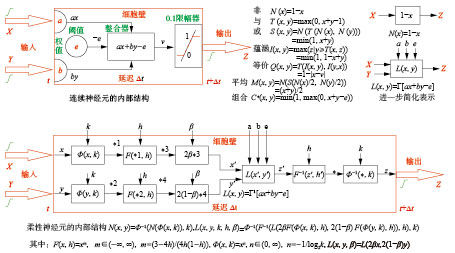Download: 图 15 柔性神经元模型 Fig. 15 Flexible neuron model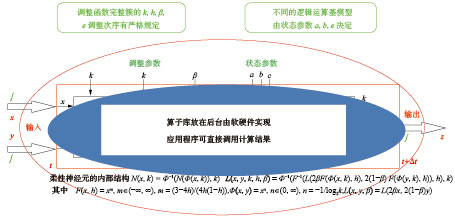Download: 图 16 完整的命题级算子库 Fig. 16 A complete library of propositional operators

2)应用程序使用算子库的两者方式。正向使用算子库的方式是：已知处理对象的因素空间关系网络 $W = \left\{ {{w_i}|i = 0,{\rm{ }}1,{\rm{ }}2,{\rm{ }}3,{\rm{ }} \cdots ,{\rm{ }}n} \right\}$ ，其中每一个 ${w_i}$ 都是一个柔性逻辑推理结点或者柔性信息变换的神经元，全部具有算子簇类型属性编码 $\left\langle {a_i},\;{b_i},\;{e_i} \right\rangle$ 和不确定性程度属性编码 $\left\langle {k_i},{h_i},{\beta _i},{\rm{ }}{e_i} \right\rangle$ ，根据这些编码参数可从算子库中准确选择相应的算子进行计算，形成精确的结果数据集合 $S = \left\{ {{s_i}|{\rm{ }}i = 0,{\rm{ }}1,{\rm{ }}2,{\rm{ }}3,{\rm{ }} \cdots ,{\rm{ }}n} \right\}$ ，反馈给应用程序使用。

5 下一步的研究工作 1) 柔性命题逻辑在生成其他逻辑中的作用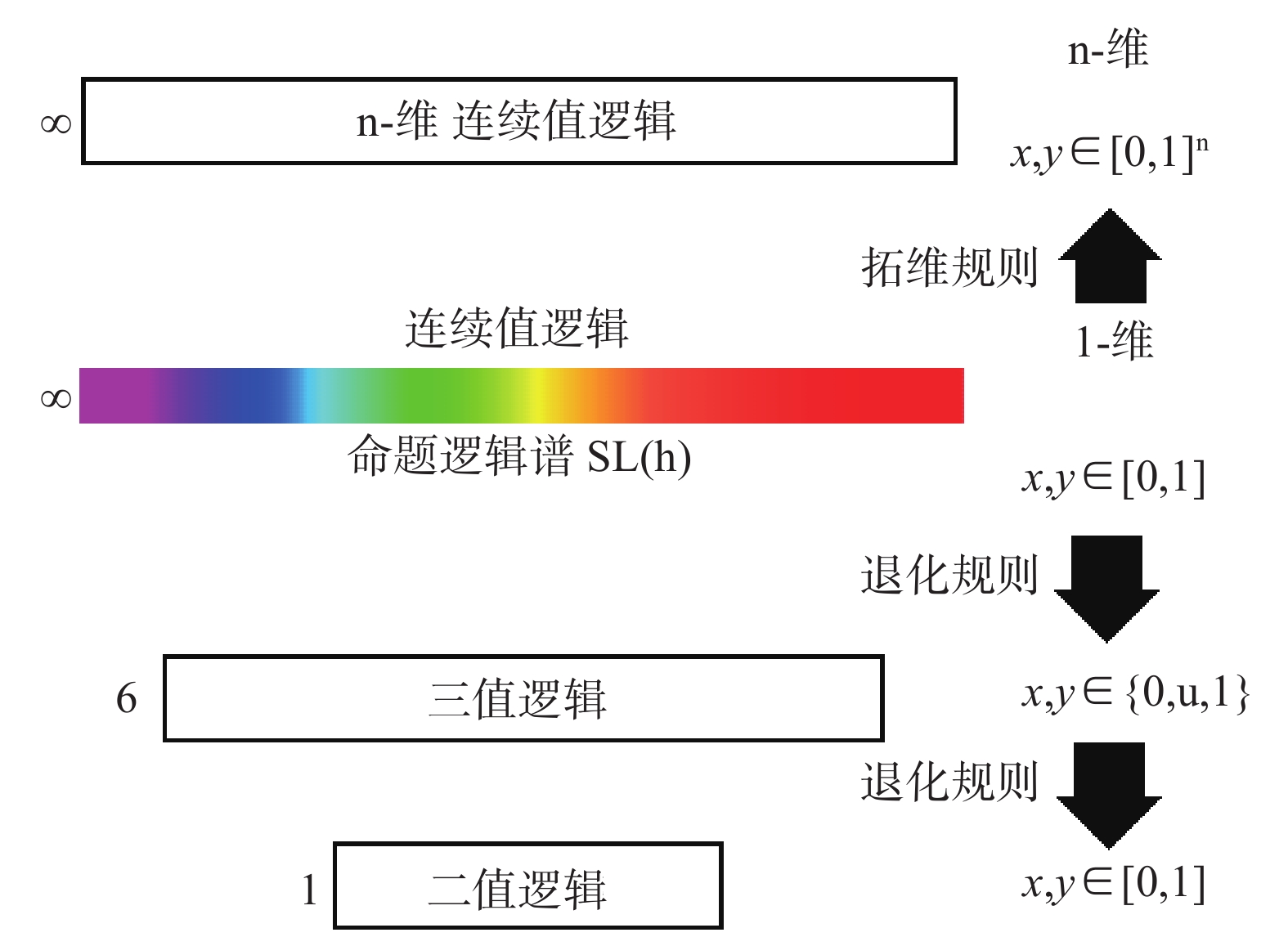Download: 图 17 生成其他逻辑的有关规则 Fig. 17 Rules for generating other logic

 ${\mathit{\boldsymbol{L}}^2}\left( {\mathit{\boldsymbol{x}},\mathit{\boldsymbol{y}},\mathit{\boldsymbol{k}},\mathit{\boldsymbol{h}},\mathit{\boldsymbol{\beta }}} \right) = \left\langle L({x_1},{y_1},k,h,\beta ),L({x_2},{y_2},k,h,\beta ) \right\rangle$

 $\begin{array}{c}{\mathit{\boldsymbol{L}}^3}\left( {\mathit{\boldsymbol{x}},\mathit{\boldsymbol{y}},\mathit{\boldsymbol{k}},\mathit{\boldsymbol{h}},\mathit{\boldsymbol{\beta }}} \right) = \langle L\left( {{x_1},{y_1},k,h,\beta } \right),\\L\left. {\left( {{x_2},{y_2},k,h,\beta } \right)} \right),L\left( {{x_3},{y_3},k,h,\beta } \right) \rangle \end{array}$

2) 柔性命题逻辑 ${\mathit{\boldsymbol{L}}}\left( {\mathit{\boldsymbol{x}},{\mathit{\boldsymbol{ y}}},{\mathit{\boldsymbol{k}}},{\mathit{\boldsymbol{h}}}} \right)$ 中可能包含更多的非标准逻辑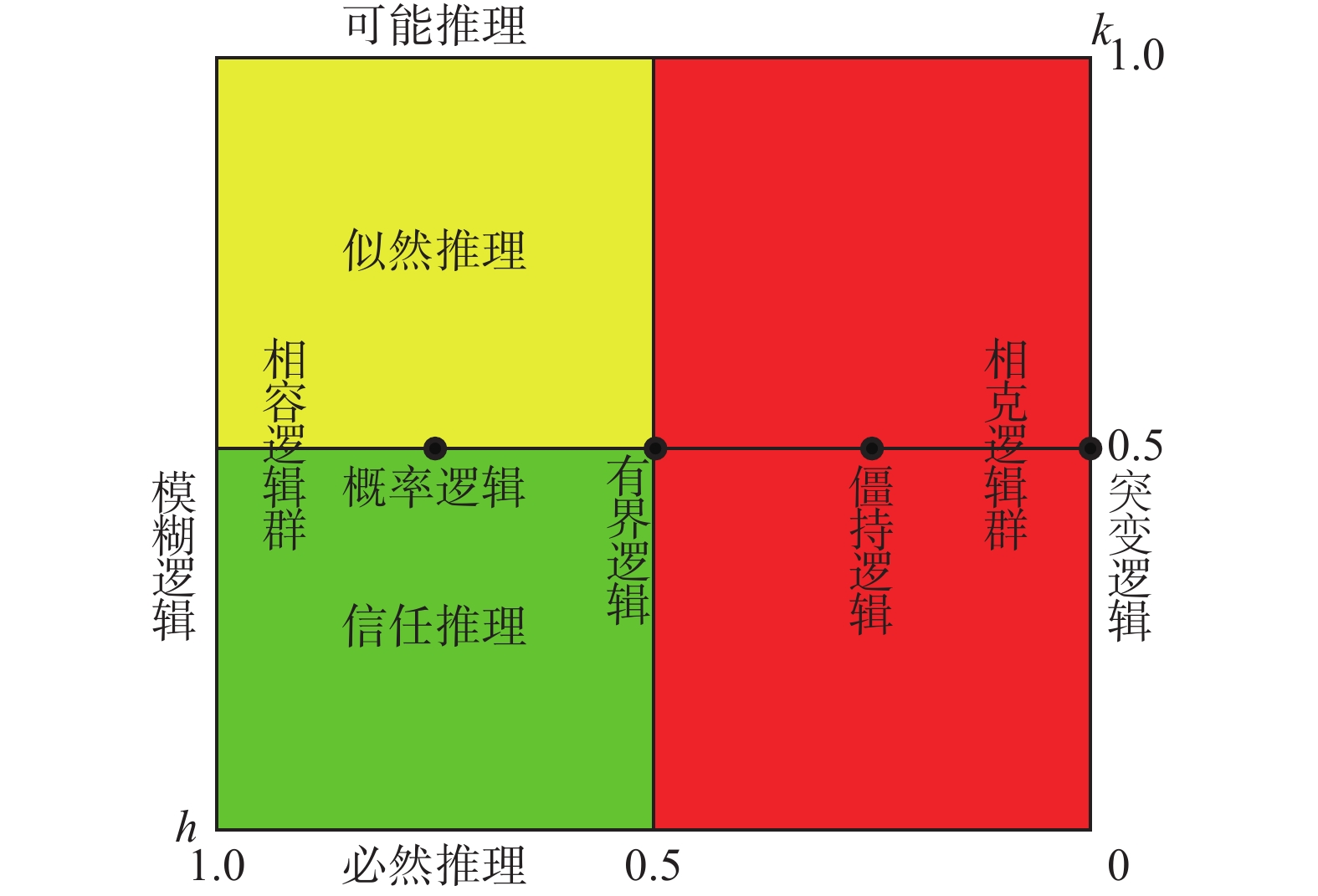Download: 图 18 需要深入研究的逻辑 Fig. 18 The logic needs to be studied in depth
3) 在柔性命题逻辑基础上建立柔性谓词逻辑，实现辩证逻辑的数学化

6 结束语

  何华灿. 人工智能导论[M]. 西安: 西北工业大学出版社, 1988: 1–4. (0)  涂序彦. 人工智能: 回顾与展望[M]. 北京: 科学出版社, 2006: 77–111. TU Xuyan. Artificial intelligence: review and prospect[M]. Beijing: Science Press, 2006: 77–111. (0)  钟义信. 高等人工智能原理: 观念·方法·模型·理论[M]. 北京: 科学出版社, 2014. (0)  GABBAY D M, GUENTHNER F. Handbook of philosophical logic[M]. 2nd ed. 2001: 1–10. (0)  陈波. 从人工智能看当代逻辑学的发展[J]. 中山大学学报论丛: 社会科学版, 2000, 20(2): 10-19. CHEN Bo. Artificial intelligence and contemporary logic study[J]. Supplement to the journal of sun Yatsen university, 2000, 20(2): 10-19. (0)  何华灿, 何智涛. 对智能科学逻辑基础研究的战略思考[J]. 智能技术, 2006, 1(2): 53-62. HE Huacan, HE Zhitao. Strategic thinking on the basic research of intelligent science logic[J]. Smart technology, 2006, 1(2): 53-62. (0)  何华灿, 刘永怀, 何大庆. 经验性思维中的泛逻辑[J]. 中国科学(D辑), 1996, 39(3): 225-234. HE Huacan, LIU Yinghuai, HE Daqing. Generalized logic in experience thinking[J]. Science in China series e-technological sciences, 1996, 39(3): 225-234. (0)  何华灿. 泛逻辑学原理[M]. 北京: 科学出版社, 2001: 1–18. HE Huacan. Universal logics principle[M]. Beijing: Science Press, 2001: 1–18. (0)  HE Huacan. Principle of universal logics[M]. Beijing: Science Press, 2006: 1–20. (0)  BÉZIAU J Y. From paraconsistent logic to universal logic[J]. Sorites, 2001(12): 5-32. (0)  BEZIAU J Y. Logica universalis-towards a general theory of logic[M]. Basel: Birkhauser Verlag, 2005: 10–16. (0)  HE Huacan. The outline on continuous-valued logic algebra[J]. International journal of advanced intelligence, 2012, 4(1): 1-30. (0)  盛骤, 谢式千, 潘承毅. 概率论与数理统计[M]. 4版. 北京: 高等教育出版社, 2008. SHENG Zhou, XIE Shiqian, PAN Chengyi. Probability and mathematical mtatistics[M]. 4th ed. Beijing: Higher Education Press, 2008. (0)  汪培庄. 因素空间与概念描述[J]. 软件学报, 1992, 3(1): 30-40. WANG Peizhuang. Factor space and description of concepts[J]. Journal of software, 1992, 3(1): 30-40. (0)  汪培庄, 李洪兴. 知识表示的数学理论[M]. 天津: 天津科学技术出版社, 1994: 1–12. (0)  刘增良. 因素神经网络理论[M]. 北京: 北师大出版社, 1990: 7–13. (0)  钟义信. 信息科学与技术导论[M]. 3版. 北京: 北京邮电大学出版社, 2015: 15–22. (0)  ZHONG Yixin. The law of " information conversion and intelligence creation[M]//BURGIN M, HOFKIRCHNER W. Information Studies and the Quest for Transdisciplinarity. Singapore: World Scientific, 2017: 165–190. (0)  ZHONG Yixin. A theory of semantic information[J]. China communications, 2017, 14(1): 1-17. (0)  何华灿. 智能论-关于人脑和其它各种系统中信息处理规律的科学[J]. 人工智能学报, 1982, 1(3): 1-18. HE Huacan. Intelligence-the science of information processing laws in the human brain and other systems[J]. Journal of artificial intelligence, 1982, 1(3): 1-18. (0)  [比]伊利亚·普里戈金. 确定性的终结——时间、混沌与新自然法则[M]. 湛敏, 译. 上海: 上海科技教育出版社, 1998: 1–12. (0)  莫绍揆. 数理逻辑概貌[M]. 北京: 科学技术文献出版社, 1989: 45–56. (0)  MCCULLOCH W S, PITTS W. A logical calculus of the ideas immanent in nervous activity[J]. Bulletin of mathematical biophysics, 1943, 5: 115-133. DOI:10.1007/BF02478259 (0)  MINSKY M L, PAPERT S. Perceptron[M]. Cambridge: MIT Press, 1969: 1–8. (0)  王元元. 计算机科学中的逻辑[M]. 北京: 科学出版社, 1989: 1–25. (0)  刘永怀. 基于广义范数的不确定性推理理论研究[D]. 西安: 西北工业大学, 1996: 20–34. LIU Yonghuai. Research on the uncertainty reasoning based on generalized norm[D]. Xi’an: Northwestern Polytechnical University, 1996: 20–34. (0)  贾澎涛. 基于柔性逻辑的时间序列数据挖掘研究[D]. 西安: 西北工业大学, 2008: 54–62. JIA Peng. Research on time series data mining based on flexible logic[D]. Xi’an: Northwestern Polytechnical University, 2008: 54–62. (0)  陈佳林. 柔性逻辑的健全性研究与应用[D]. 北京: 北京邮电大学, 2011: 1–21. CHEN Jialin. The study and application of flexible logic[D]. Beijing: Beijing University of Posts and Telecommunications, 2011: 1–21. (0)  周红军. 计量逻辑及其应用[M]. 北京: 科学出版社, 2015: 2–12. (0)  祝峰, 何华灿. 粗集的公理化[J]. 计算机学报, 2000, 23(3): 330-333. ZHU Feng, HE Huacan. The axiomatization of the rough set[J]. Chinese journal of computers, 2000, 23(3): 330-333. (0)  张文修. 不确定性推理原理[M]. 西安: 西安交通大学出版社, 1996: 36–64. (0)  何华灿, 何智涛, 王华. 论第2次数理逻辑革命[J]. 智能系统学报, 2006, 1(1): 29-37. HE Huacan, HE Zhitao, WANG Hua. On the second revolution of mathematical logic[J]. CAAI transactions on intelligent systems, 2006, 1(1): 29-37. (0)